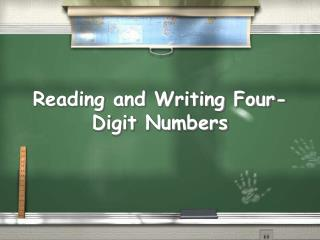DownloadDownload Presentation# Reading and Writing Four-Digit Numbers

Download Presentation## Reading and Writing Four-Digit Numbers

- - - - - - - - - - - - - - - - - - - - - - - - - - - E N D - - - - - - - - - - - - - - - - - - - - - - - - - - -
##### Presentation Transcript

1. Reading and Writing Four-Digit Numbers

2. Expanded Form 2,000 + 300 + 70 + 5

3. Standard Form 2,375

4. Word name Two thousand, three hundred, seventy-five

5. Write the number in standard form. 4,000 + 600 + 20 + 5 4,625

6. Write the number in standard form. Five thousand, forty-eight 5,048

7. Write the number in standard form. Three thousand, seven hundred five 3,705

8. Write the number in standard form. 8,000 + 500 + 70 + 7 8,577

9. Write the number in standard form. Seven thousand, thirty 7,030

10. Write the number in standard form. 6,000 + 900 + 50 6,950

11. Write the number in expanded form. 3,324 3,000 + 300 + 20 + 4

12. Write the number in expanded form. 650 600 + 50

13. Write the number in expanded form. 1,746 1,000 + 700 + 40 + 6

14. Write the number in expanded form. 9,400 9,000 + 400

15. Write the number in expanded form. 2,946 2,000 + 900 + 40 + 6

16. Write the number in expanded form. 335 300 + 30 + 5

17. Write the number in expanded form. 4,806 4,000 + 800 + 6

18. Write the number in expanded form. 3,006 3,000 + 6